# [APPL] What happens if we invest \$ 1,000 in US Apple annually?

## [APPL] What happens if we invest \$ 1,000 in US Apple annually?

I use iphone.

I also buy songs at itunes store and charge for the app.

Recently apple has increased dividends every year.

I will continue to hold this stock for a long time.

I calculated the case of \$ 1,000 regular investment in Apple each year (dividends are reinvested).

## Calculation condition

I calculated how much the value of stock would be if we invest \$ 1,000 in US Apple annually.

I calculated it based on the past stock price and the dividend per share.

The regular investment period is from 2003 to 2018, from 2008 to 2018, and from 2013 to 2018.

I calculated the case of reinvesting the dividend and the case of not reinvesting it.

The timing to invest \$ 1,000 is the first trading day of each year.

The timing for investing \$ 1,000 is the first trading day before the Ex-dividend date of each year.

Suppose we can buy the stock at the closing price.

The dividend is paid on the Payment dividened date.

Suppose that we can buy the stock at the closing price on the Payment dividened date.

Taxes and fees are not considered.

In the year with no dividend,suppose that we buy at the closing price on the first day of trading in that year.

## Calculation result

### The case of investing \$ 1,000 annually (dividends are reinvested) from 2005 to 2018

Table 1 and Figure 1 show the calculation results of holding stock value when \$ 1,000 is invested annually (dividends are reinvested) from 2003 to 2018.

It will be \$ 125,160 in November 2018.

In the case of not reinvesting, it will be \$ 111,190 in November 2018.

If we save \$ 1,000 every year from 2005, we will save \$ 14,000.

It was found that investing (dividends are reinvested) can increase assets approximately 9 times more than saving money.

Table 1  the calculation results of holding stock value when \$ 1,000 is invested annually (dividends are reinvested) from 2003 to 2018.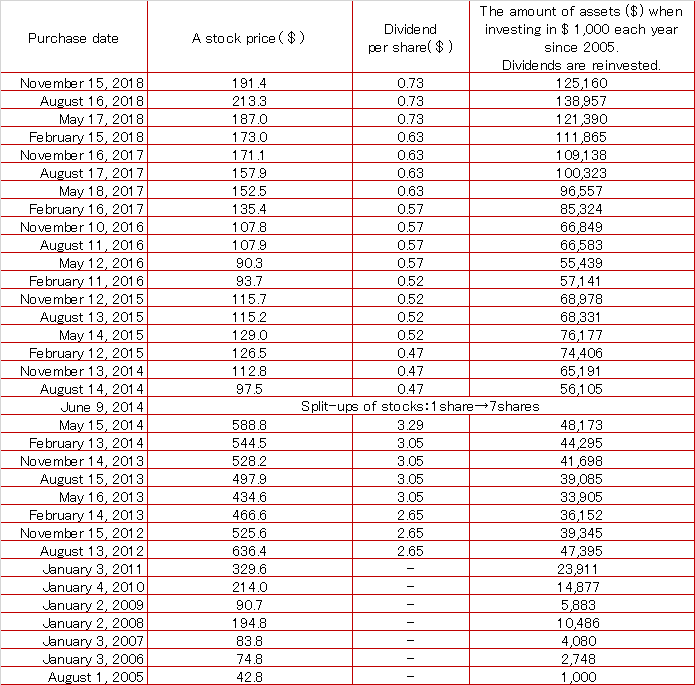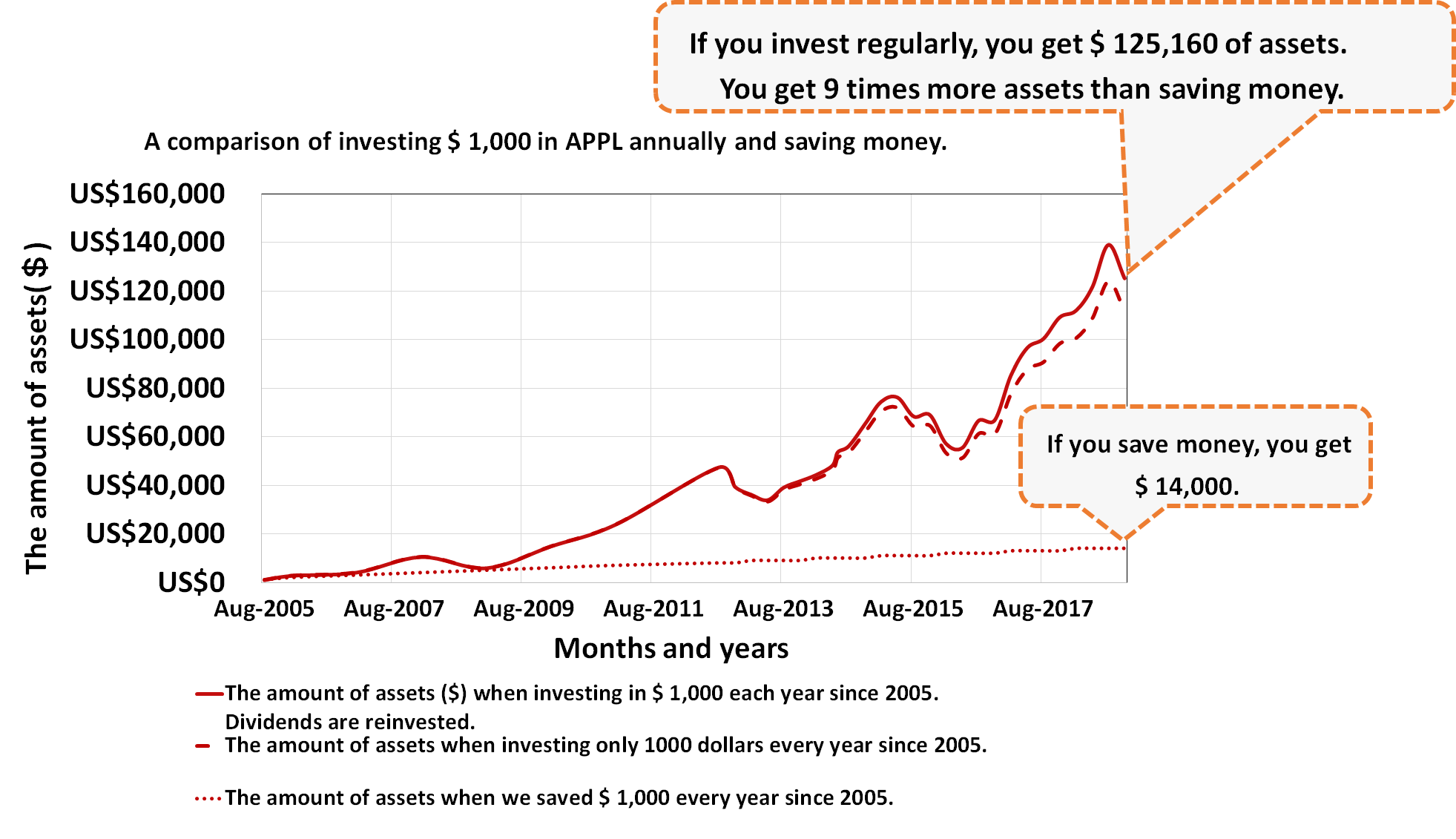Figure 1  A comparison of investing \$ 1,000 in APPL annually and saving money.

### The case of investing \$ 1,000 annually (dividends are reinvested) from 2008 to 2018

Table 2 and Figure 2 show the calculation results of holding stock value when \$ 1,000 is invested annually (dividends are reinvested) from 2008 to 2018.

It will be \$ 84,769 in November 2018.

In the case of not reinvesting, it will be \$ 75,762 in November 2018.

If we save \$ 1,000 every year from 2008, we will save \$ 11,000.

It was found that investing (dividends are reinvested) can increase assets approximately 8 times more than saving money.

Table 2  the calculation results of holding stock value when \$ 1,000 is invested annually (dividends are reinvested) from 2008 to 2018.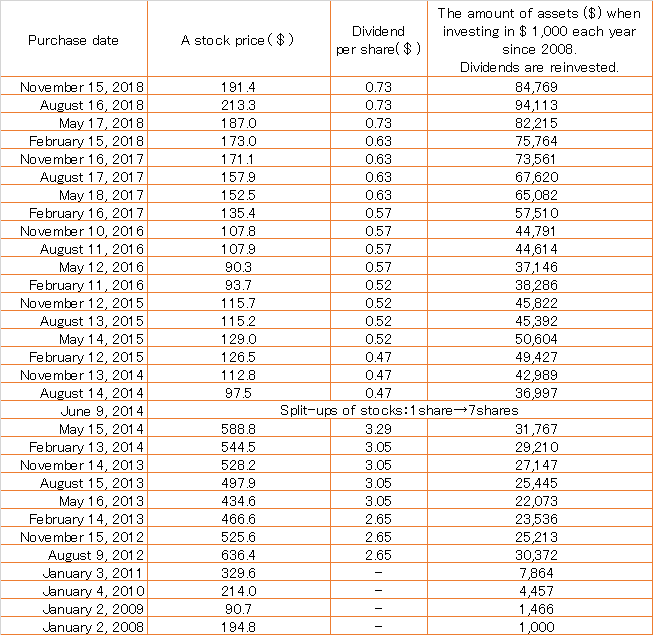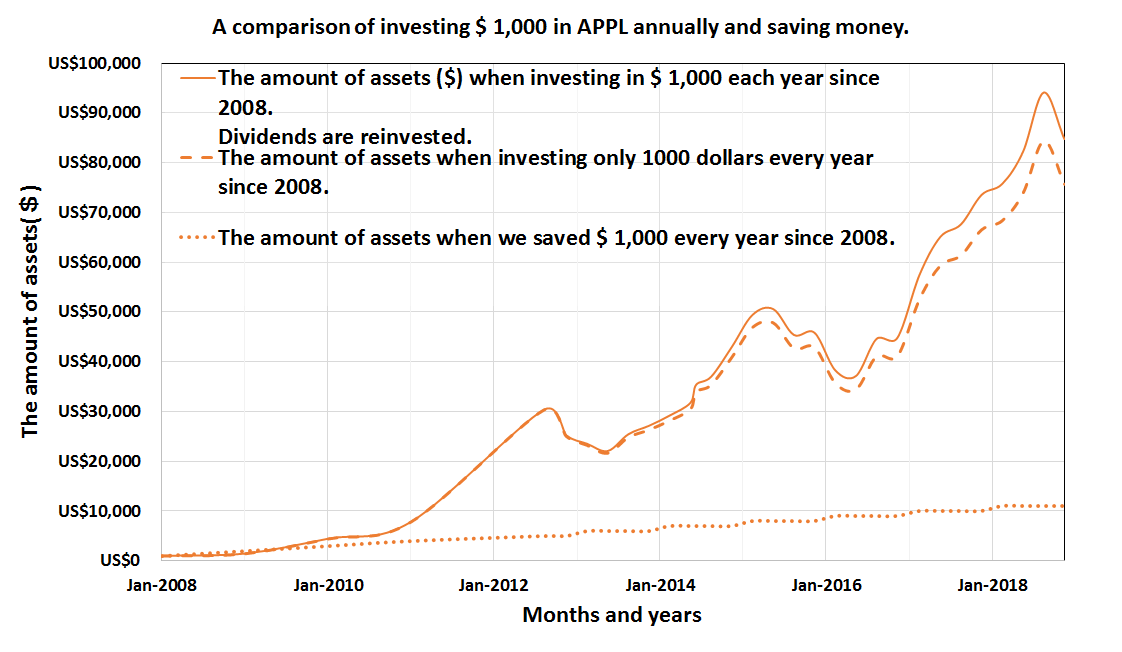Figure 2  A comparison of investing \$ 1,000 in APPL annually and saving money.

### The case of investing \$ 1,000 annually (dividends are reinvested) from 2013 to 2018

Table 3 and Figure 3 show the calculation results of holding stock value when \$ 1,000 is invested annually (dividends are reinvested) from 2013 to 2018.

It will be \$ 12,703 in November 2018.

In the case of not reinvesting, it will be \$ 11,814 in November 2018.

If we save \$ 1,000 every year from 2013, we will save \$ 6,000.

It was found that investing (dividends are reinvested) can increase assets approximately 8 times more than saving money.

Table 3  the calculation results of holding stock value when \$ 1,000 is invested annually (dividends are reinvested) from 2013 to 2018.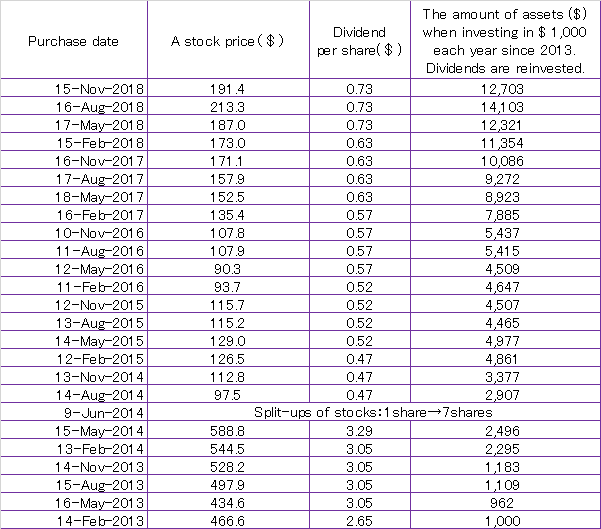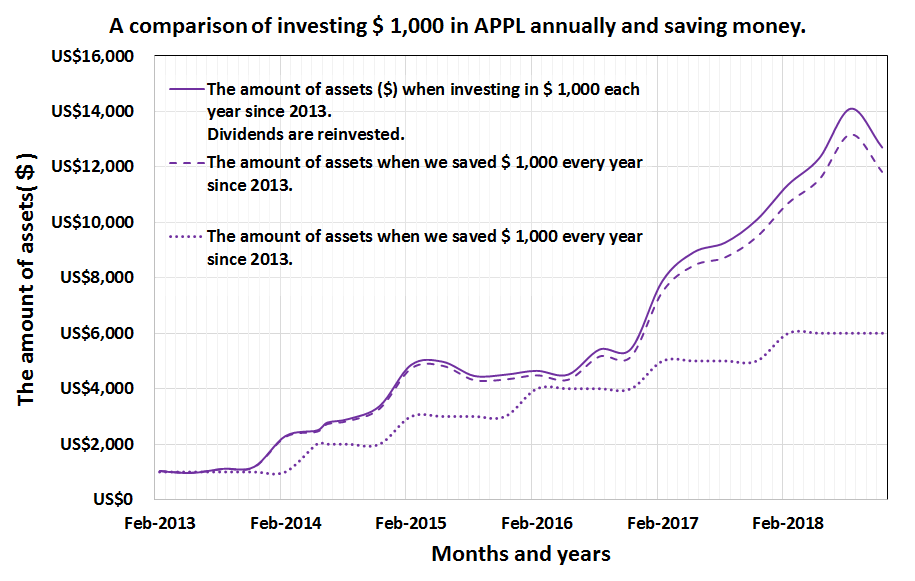Figure 3  A comparison of investing \$ 1,000 in APPL annually and saving money.

### It summarized the calculation results in one graph.

Figure 4 summarizes the calculation results for each period.

It was found that the value of stock holding is the largest when the regular investment (dividends are reinvested) is started from 2005.

Contributing factors are the continuous increase in dividend, reinvestment of dividends and rise in stock prices.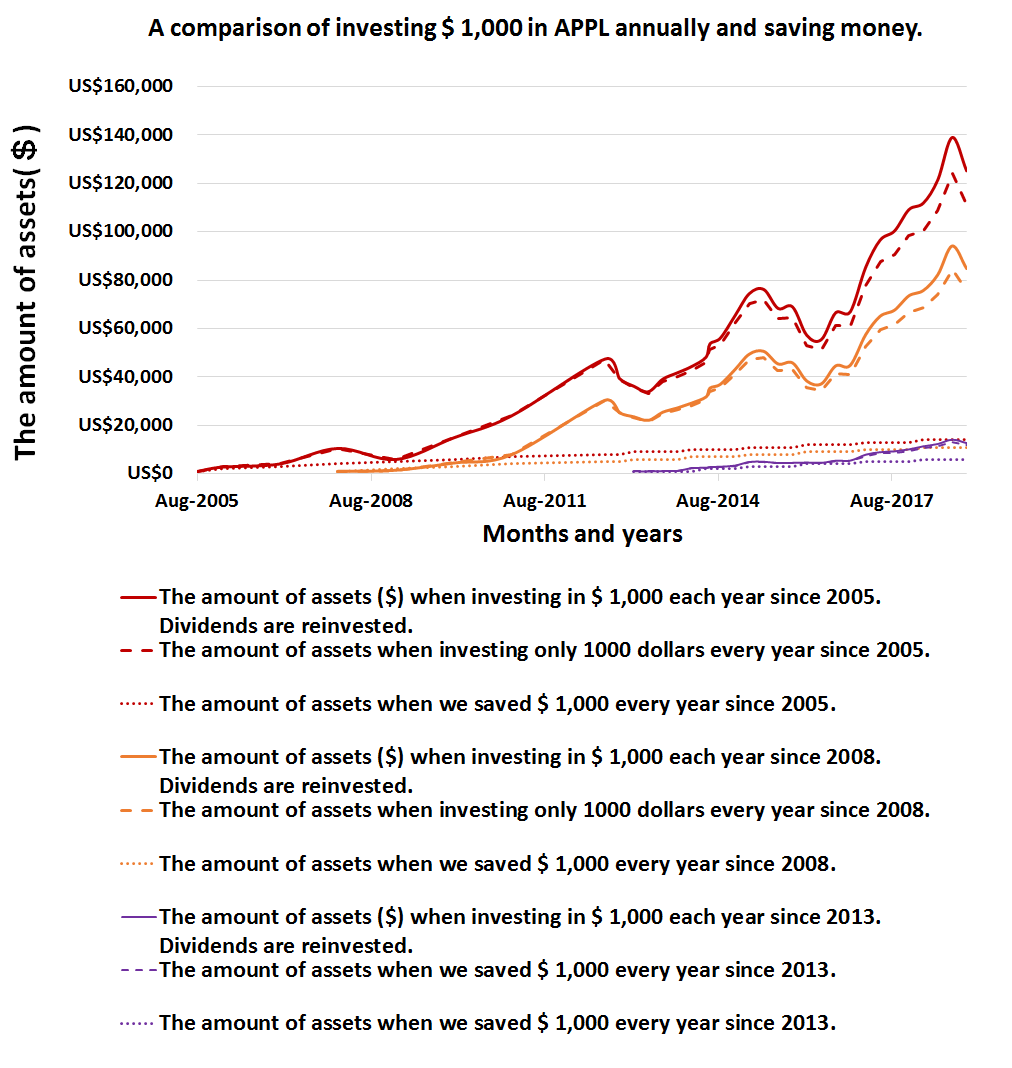Figure 4  calculation results are summarized.

### Dividends that can be received if we invest \$ 1,000 annually (dividends are reinvested) from 2005 to 2018.

Table 4 and Figure 5 show the dividends that can be received when investing (dividends are reinvested) from 2005.

In 2018, it was found that we could receive about \$ 1,800 in a year.

Table 4  Dividends received from investment in 2005 (dividend reinvestment).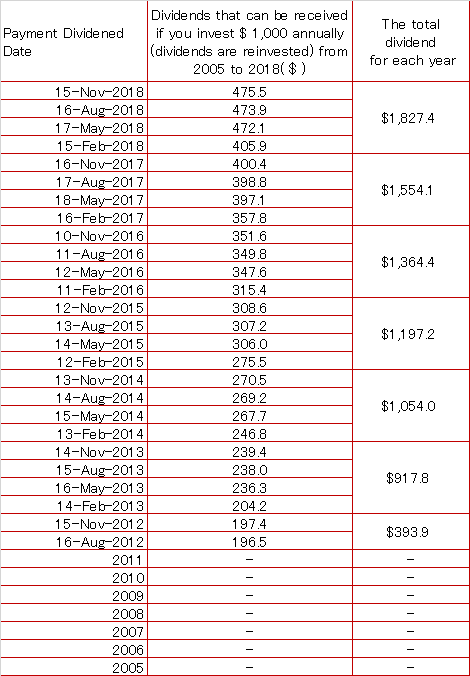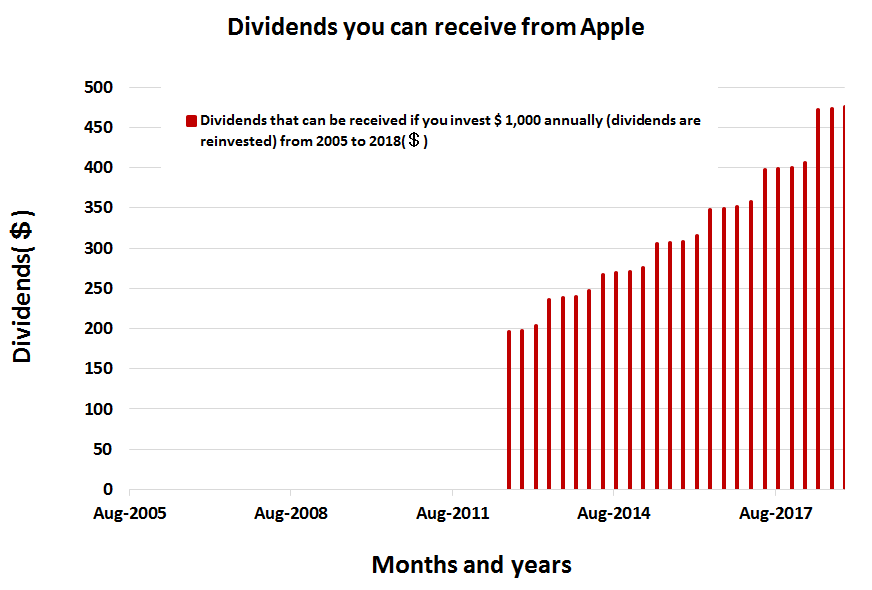Figure 5  Dividends received when investing from 2005 (dividends are reinvested).